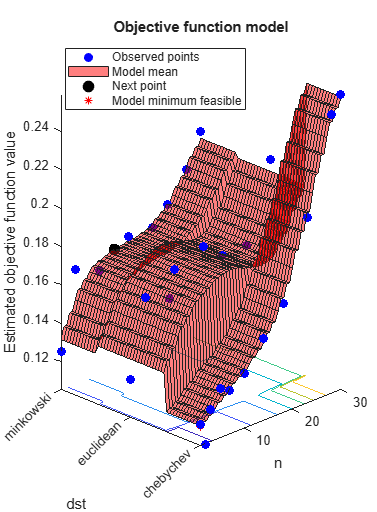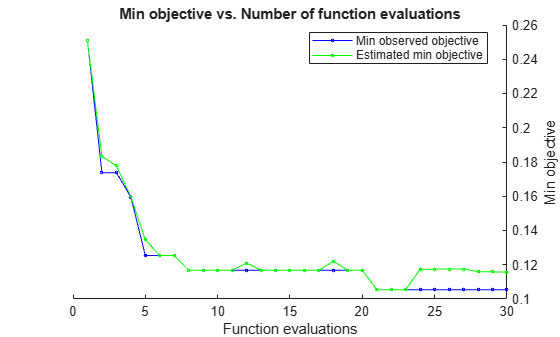# bestPoint

Best point in a Bayesian optimization according to a criterion

## Syntax

``x = bestPoint(results)``
``x = bestPoint(results,Name,Value)``
``````[x,CriterionValue] = bestPoint(___)``````
``````[x,CriterionValue,iteration] = bestPoint(___)``````

## Description

example

````x = bestPoint(results)` returns the best feasible point in the Bayesian model `results` according to the default criterion `'min-visited-upper-confidence-interval'`.```

example

````x = bestPoint(results,Name,Value)` modifies the best point using name-value pairs.```

example

``````[x,CriterionValue] = bestPoint(___)```, for any previous syntax, also returns the value of the criterion at `x`.```

example

``````[x,CriterionValue,iteration] = bestPoint(___)``` also returns the iteration number at which the best point was returned. Applies when the `Criterion` name-value pair is `'min-observed'`, `'min-visited-mean'`, or the default `'min-visited-upper-confidence-interval'`.```

## Examples

collapse all

This example shows how to obtain the best point of an optimized classifier.

Optimize a KNN classifier for the `ionosphere` data, meaning find parameters that minimize the cross-validation loss. Minimize over nearest-neighborhood sizes from 1 to 30, and over the distance functions `'chebychev'`, `'euclidean'`, and `'minkowski'`.

For reproducibility, set the random seed, and set the `AcquisitionFunctionName` option to `'expected-improvement-plus'`.

```load ionosphere rng(11) num = optimizableVariable('n',[1,30],'Type','integer'); dst = optimizableVariable('dst',{'chebychev','euclidean','minkowski'},'Type','categorical'); c = cvpartition(351,'Kfold',5); fun = @(x)kfoldLoss(fitcknn(X,Y,'CVPartition',c,'NumNeighbors',x.n,... 'Distance',char(x.dst),'NSMethod','exhaustive')); results = bayesopt(fun,[num,dst],'Verbose',0,... 'AcquisitionFunctionName','expected-improvement-plus');```Obtain the best point according to the default `'min-visited-upper-confidence-interval'` criterion.

`x = bestPoint(results)`
```x=1×2 table n dst _ _________ 1 chebychev ```

The lowest estimated cross-validation loss occurs for one nearest neighbor and `'chebychev'` distance.

Careful examination of the objective function model plot shows a point with two nearest neighbors and `'chebychev'` distance that has a lower objective function value. Find this point using a different criterion.

`x = bestPoint(results,'Criterion','min-observed')`
```x=1×2 table n dst _ _________ 2 chebychev ```

Also find the minimum observed objective function value, and the iteration number at which it was observed.

`[x,CriterionValue,iteration] = bestPoint(results,'Criterion','min-observed')`
```x=1×2 table n dst _ _________ 2 chebychev ```
```CriterionValue = 0.1054 ```
```iteration = 21 ```

## Input Arguments

collapse all

Bayesian optimization results, specified as a `BayesianOptimization` object.

### Name-Value Arguments

Specify optional pairs of arguments as `Name1=Value1,...,NameN=ValueN`, where `Name` is the argument name and `Value` is the corresponding value. Name-value arguments must appear after other arguments, but the order of the pairs does not matter.

Before R2021a, use commas to separate each name and value, and enclose `Name` in quotes.

Example: ```x = bestPoint(results,'Criterion','min-observed')```

Best point criterion, specified as the comma-separated pair consisting of `'Criterion'` and a criterion name. The names are case-insensitive, do not require `-` characters, and require only enough characters to make the name uniquely distinguishable.

Criterion NameMeaning
`'min-observed'``x` is the feasible point with minimum observed objective.
`'min-mean'``x` is the feasible point where the objective model mean is minimized.
`'min-upper-confidence-interval'``x` is the feasible point minimizing an upper confidence interval of the objective model. See `alpha`.
`'min-visited-mean'``x` is the feasible point where the objective model mean is minimized among the visited points.
`'min-visited-upper-confidence-interval'``x` is the feasible point minimizing an upper confidence interval of the objective model among the visited points. See `alpha`.

Example: `'Criterion','min-visited-mean'`

Probability that the modeled objective mean exceeds `CriterionValue`, specified as the comma-separated pair consisting of `'alpha'` and a scalar between `0` and `1`. `alpha` relates to the `'min-upper-confidence-interval'` and `'min-visited-upper-confidence-interval'` `Criterion` values. The definition for the upper confidence interval is the value `Y` where

P(meanQ(`fun`(`x`)) > `Y`) = `alpha`,

where `fun` is the objective function, and the mean is calculated with respect to the posterior distribution Q.

Example: `'alpha',0.05`

Data Types: `double`

## Output Arguments

collapse all

Best point, returned as a `1`-by-`D` table, where D is the number of variables. The meaning of “best” is with respect to `Criterion`.

Value of criterion, returned as a real scalar. The value depends on the setting of the `Criterion` name-value pair, which has a default value of `'min-visited-upper-confidence-interval'`.

Criterion NameMeaning
`'min-observed'`Minimum observed objective.
`'min-mean'`Minimum of model mean.
`'min-upper-confidence-interval'`Value `Y` satisfying the equation P(meanQ(`fun`(`x`)) > `Y`) = `alpha`.
`'min-visited-mean'`Minimum of observed model mean.
`'min-visited-upper-confidence-interval'`Value `Y` satisfying the equation P(meanQ(`fun`(`x`)) > `Y`) = `alpha` among observed points.

Iteration number at which best point was observed, returned as a positive integer. The best point is defined by `CriterionValue`.

## Version History

Introduced in R2016b